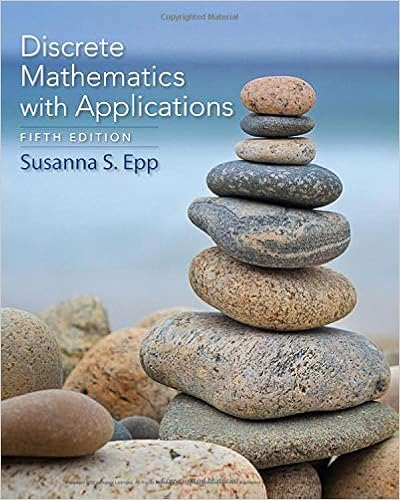# Margin of the contingency table frequency marginal

• Notes
• 28

This preview shows page 9 - 13 out of 28 pages.

##### We have textbook solutions for you!
The document you are viewing contains questions related to this textbook.The document you are viewing contains questions related to this textbook.
Chapter 4 / Exercise 3
Discrete Mathematics With Applications
EppExpert Verified
marginof the contingency table.)Frequencymarginal distribution, just add up all the numbers in each row and column.The total in the bottom right corner is the totalnumber of individuals.Relative Frequencymarginal distribution, take the sums you calculated in the frequency marginal distribution, and divide each one by the totalnumber of individuals (218,014)The bottom rowwith the relative frequencies should add up to 1 (or approximately 1). The right columnwith the relative frequencies should add up to 1 (or approximately 1).The relative frequency distribution lets us see what percentage of people are employed/unemployed/ not in the labor force. And it lets us see what percent of people have each education level.Conditional Distributionlists the relative frequency of each category of the response variable, given a
##### We have textbook solutions for you!
The document you are viewing contains questions related to this textbook.The document you are viewing contains questions related to this textbook.
Chapter 4 / Exercise 3
Discrete Mathematics With Applications
EppExpert Verified
specific value of the explanatory variable in the contingency table.So here was the original frequency contingency table.We are treating the Level of Education as the Explanatory variable because we want to know “How doeseducationlevel affectemployment?”. Each level of education is a column, so we will find therelative frequency distribution of each column.In each column, the relative frequencies should add to 1.Now we can see that the answer to “What percentage of people who have a bachelor’s degree or higher are employed?” is 70.5% and “What percentage of people who are high school graduates are employed?” is 50% and we can make conclusions like “People with Bachelor’s degrees are more likely to be employed than people who are just high school graduates”.
PROBABILITYProbability-Describes how likely it is that some event will happen. The long-term proportion in which a certain outcome is observed is the probabilityof that outcome.Experiment- Any process with uncertain results that can be repeated.Outcome-The result of one trial of a probability experiment.The Sample Space-A list of all possible outcomes.Event-Consists of one outcome or more outcomes.PROBABILITY PROPERTIESProbability must always be between 0 and 1!A probability of 0 means a 0% chance of happening (IMPOSSIBLE)A probability of 1 means a 100% chance of happening (CERTAINTY)The sum of probabilities for all possible outcomes must equal 1.PROBABILITY MODEL Probability Model-Lists the possible outcomes of a probability experiment and each outcome probability. A probability model must satisfy the probability properties listed above.Unusual Event-An event that has a low probability of occurring. Typically, an event with a probability less than 0.05 (or 5%) is considered unusual, but this is not set in stone The researcher and the context of the problem determine the probability that separates unusual events from not so unusual events.CLASSICAL VS EMPIRICAL PROBABILITY There are three ways to determine the probability of an event.
Classical ProbabilityIf the outcomes are equally likely, you can calculate the probability of an event by counting the number of ways an
•••